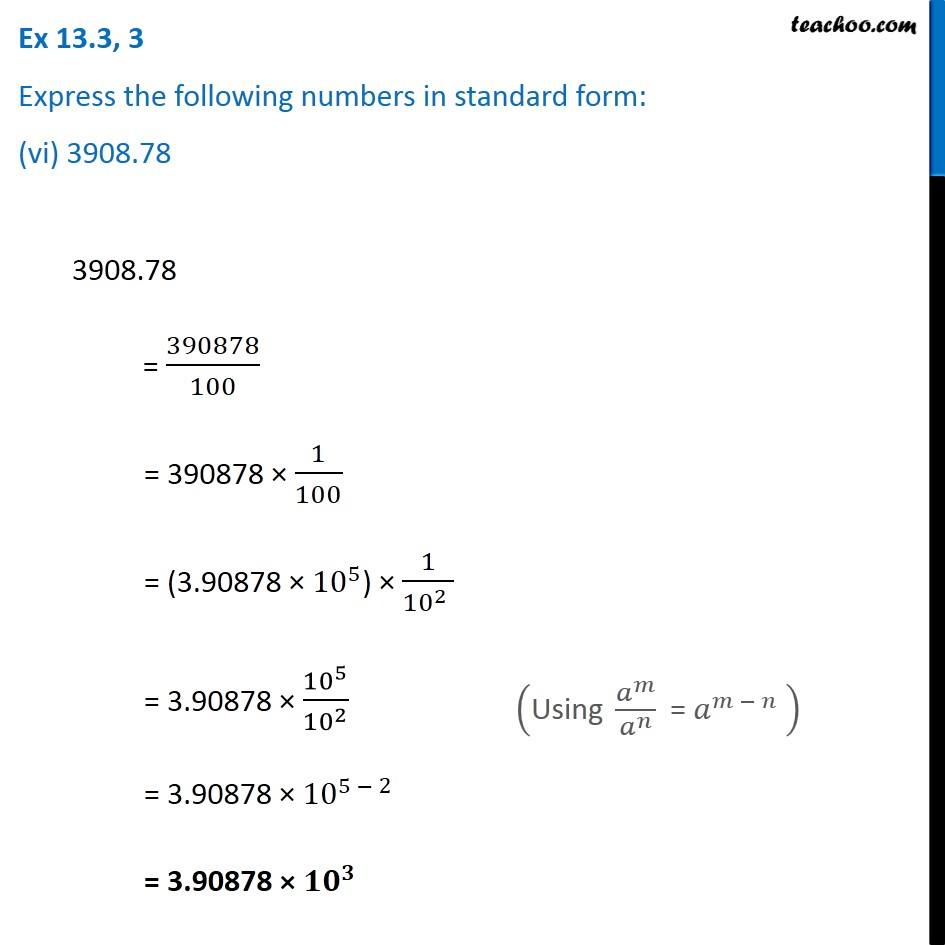Ex 13.3

Chapter 13 Class 7 Exponents and Powers
Serial order wiseGet live Maths 1-on-1 Classs - Class 6 to 12

### Transcript

Ex 13.3, 3 Express the following numbers in standard form: (vi) 3908.78 3908.78 = 390878/100 = 390878 × 1/100 = (3.90878 × 〖10〗^5) × 1/(〖10〗^2 ) = 3.90878 × 〖10〗^5/〖10〗^2 = 3.90878 × 〖10〗^(5 − 2) = 3.90878 × 〖𝟏𝟎〗^𝟑 ("Using " 𝑎^𝑚/𝑎^𝑛 " = " 𝑎^(𝑚 − 𝑛) " " )